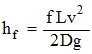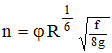The Darcy Equation is a theoretical equation that predicts the frictional energy loss in a pipe based on the velocity of the fluid and the resistance due to friction. It is used almost exclusively to calculate head loss due to friction in turbulent flow.Where:

f = Darcy resistance factor

L = Length of the pipe

D = Pipe diameter

V = Mean velocity

g = acceleration due to gravity

The Darcy friction factor, f, is usually selected from a chart known as the Moody diagram. The Moody diagram is a family of curves that relate the friction factor, f, to Reynolds number, Re, and the relative roughness of a pipe, e/D.

Alternatively, the Darcy friction factor is related to Manning's n through the following relationship:Where:

n = Manning friction factor

j = Constant of 1.00 for metric and 1.49 for English units